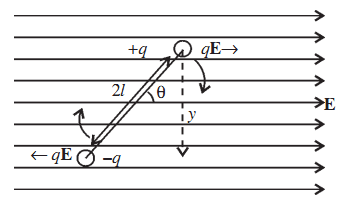# Electric Dipole in Uniform Field

A force qE acts on charge +q along the +x direction and an equal force acts on charge –q in the –x direction. Two equal, unlike and parallel forces form a couple and tend to rotate the dipole in clockwise direction. This couple tends to align the dipole in the direction of the external electric field E.τ = Force × arm of the couple

= qE × y

= qE × 2l sin θ

= pE sin θ

τ = p × E

When θ = 0, the torque is zero and for θ = 90°, the torque on the dipole is maximum, equal to pE.